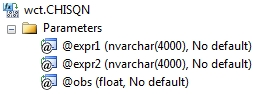# SQL Server chi-squared statistic for normalized data

CHISQN

Updated: 28 February 2011

Note: This documentation is for the SQL2008 (and later) version of this XLeratorDB function, it is not compatible with SQL Server 2005.

Use the aggregate CHISQN to calculate the chi-square (χ2) statistic for normalized tables. This function calculates the chi-square statistic by finding the difference between each observed and theoretical frequency for each possible outcome, squaring them, dividing each by the theoretical frequency, and taking the sum of the results. A second important part of determining the test statistic is to define the degrees of freedom of the test: this is essentially the number of squares errors involving the observed frequencies adjusted for the effect of using some of those observations to define the expected frequencies.

CHISQN automatically calculates the expected results.

The value of the chi-square statistic is:Where
r              is the number of rows
c              is the number of columns
O             is the Observed result
E              is the Expected result
SyntaxArguments
@Expr1
the name or number of the rows.
@Expr2
the name or number of the columns.
@Obs
the observed value. @Obs is an expression of type float or of a type that can be implicitly converted to float.
Return Types
float
Remarks
·         CHISQN is designed for normalized tables.
·         CHISQN automatically calculates the expected values. If you want to enter the expected values use the CHISQN2 function.
·         CHISQN is an aggregate function and follows the same conventions as all other aggregate functions in SQL Server.
·         If you have previously used the CHISQN scalar function, the CHISQN aggregate has a different syntax. The CHISQN scalar function is no longer available in XLeratorDB/statistics2008, though you can still use the scalar CHISQN_q.
Examples
In this hypothetical situation, we want to determine if there is an association between population density and the preference for a sport from among baseball, football, and basketball. We will use the CHISQN function to calculate the chi-square statistic.
SELECT wct.CHISQN(
sport       --@expr1
,locale     --@expr2
,result     --@obs
) as CHISQ
FROM (VALUES
('Baseball', 'Rural', 60),
('Baseball', 'Suburban', 43),
('Baseball', 'Urban', 35),
('Football', 'Rural', 52),
('Football', 'Suburban', 48),
('Football', 'Urban', 28)
) n(sport, locale, result)

This produces the following result.
CHISQ
----------------------
22.451703426585

(1 row(s) affected)

What if the data had not been normalized? We could use that following SQL to take de-normalized data (the way it might be in a spreadsheet) and turn it into normalized data.
SELECT wct.CHISQN(n.sport, x.locale, x.result) as CHISQ
FROM (VALUES
('Baseball', 60, 43, 35),
('Football', 52, 48, 28)
) n(sport, rural, suburban, urban)
CROSS APPLY(VALUES
('rural', rural),
('suburban', suburban),
('urban',urban)
) x(locale, result)

This produces the following result.
CHISQ
----------------------
22.451703426585

(1 row(s) affected)

In this example, the de-normalized table contains another column, region. We will calculate the chi-square statistic for each region.
SELECT n.region,
wct.CHISQN(n.sport, x.locale, x.result) as CHISQ
FROM (VALUES
('Midwest','Baseball',27,38,36),
('Midwest','Football',43,29,36),
('Northeast','Baseball',39,46,42),
('Northeast','Football',35,30,41),
('Southeast','Baseball',31,36,33),
('Southeast','Football',31,24,40),
('Southwest','Baseball',44,19,34),
('Southwest','Football',41,29,36),
('West','Baseball',43,33,30),
('West','Football',30,36,30)
) n(region, sport, urban, rural, suburban)
CROSS APPLY(VALUES
('rural', rural),
('suburban', suburban),
('urban',urban)
) x(locale, result)
GROUP BY region

This produces the following result.
region                     CHISQ
--------- ----------------------
Midwest         6.82237540453074
Northeast       2.88288327437734
Southeast       4.24269415109385
Southwest       11.8327601855115
West            2.05463121587102

(5 row(s) affected)

### SupportCopyright 2008-2021 Westclintech LLC         Privacy Policy        Terms of Service